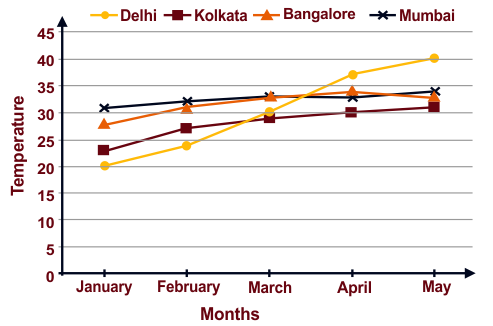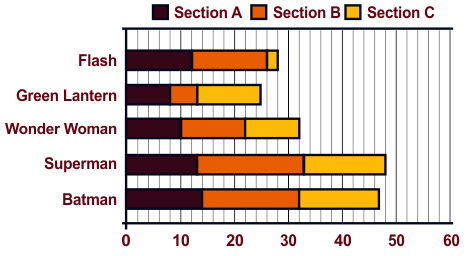# Indian Talent Olympiad - IMO PDF Sample Papers for Class 6

Class 6 sample paper & practice questions for International Maths Olympiad (IMO) level 1 are given below. Syllabus for level 1 is also mentioned for these exams. You can refer these sample paper & quiz for preparing for the exam.#### Resources:

##### Sample Questions from Olympiad Success:
 Q.1 Q.2 Q.3 Q.4 Q.5 Q.6 Q.7 Q.8 Q.9 Q.10
 Q.1 The fraction which is not equal to 4/5 is: a) 40/50 b) 12/15 c) 16/20 d) 9/15
 Q.2 Here is the average monthly temperature of the following cities. With respect to this, answer question. Which city is the hottest in May?a) Kolkata b) Bangalore c) Delhi d) Mumbai
 Q.3 In 3 sections, the children were asked their favourite superhero and the graph was plotted. Answer questions. In which section is Batman most popular?a) Section A b) Section B c) Section C d) All of these
 Q.4 3 X 10000 + 7 X 1000 + 9 X 100 + 0 X 10 + 4 is the same as: a) 37914 b) 37940 c) 37904 d) 379409
 Q.5 Number of whole number lying between -5 and 5 are: a) 10 b) 3 c) 4 d) 5
 Q.6 The lines which do not intersect and have equal distance between them are called: a) perpendicular lines b) parallel lines c) intersecting lines d) straight lines
 Q.7 If a bicycle wheel has 48 spokes, then the angle between a pair of two consecutive spokes is: a) 11/2 b) 15/2 c) 2/11 d) 2/15
 Q.8 Which property is reflected in the following? 7 x 5 = 5 x 7 a) Closure b) Associative c) Distributive d) Commutative
 Q.9 The perimeter of regular octagon is 16 cm, the length of each side will be: a) 4 cm b) 1 cm c) 2 cm d) 8 cm
 Q.10 If x takes the value 2, then the value of x + 10 is: a) 12 b) 13 c) 14 d) 15Sample PDF of Indian Talent Olympiad - International Maths Olympiad (IMO) PDF Sample Papers for Class 6:

 Q.1 )d Q.2 )c Q.3 )b Q.4 )c Q.5 )d Q.6 )b Q.7 )b Q.8 )d Q.9 )c Q.10 )a

Q.1 : d | Q.2 : c | Q.3 : b | Q.4 : c | Q.5 : d | Q.6 : b | Q.7 : b | Q.8 : d | Q.9 : c | Q.10 : a